# Selina Solutions Concise Mathematics Class 6 Chapter 22 Simple (Linear) Equations Exercise 22 (D)

Selina Solutions Concise Mathematics Class 6 Chapter 22 Simple (Linear) Equations Exercise 22(D) are created by highly experienced faculty in the education industry, as per the ICSE syllabus. Various solved examples present before each exercise help students analyse the questions that would appear in the annual exam. Those who aspire to obtain high marks are suggested to practice Selina Solutions repeatedly. In order to improve problem solving skills, students can access Selina Solutions Concise Mathematics Class 6 Chapter 22 Simple (Linear) Equations Exercise 22(D) PDF, free from the available links below.

## Selina Solutions Concise Mathematics Class 6 Chapter 22 Simple (Linear) Equations Exercise 22(D) Download PDF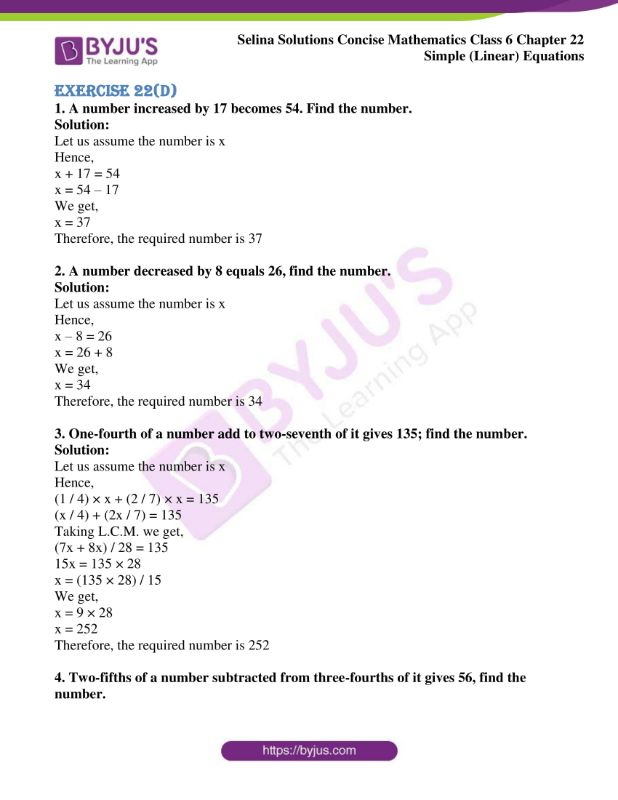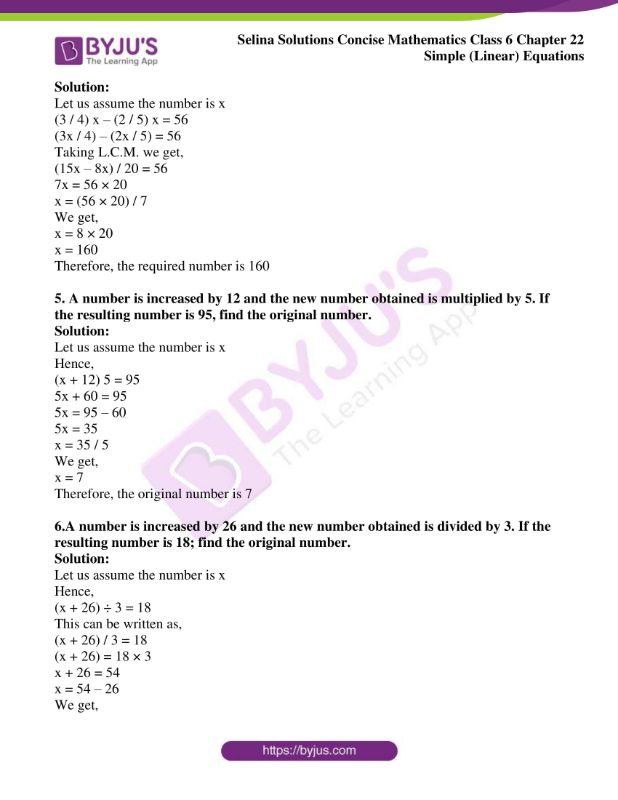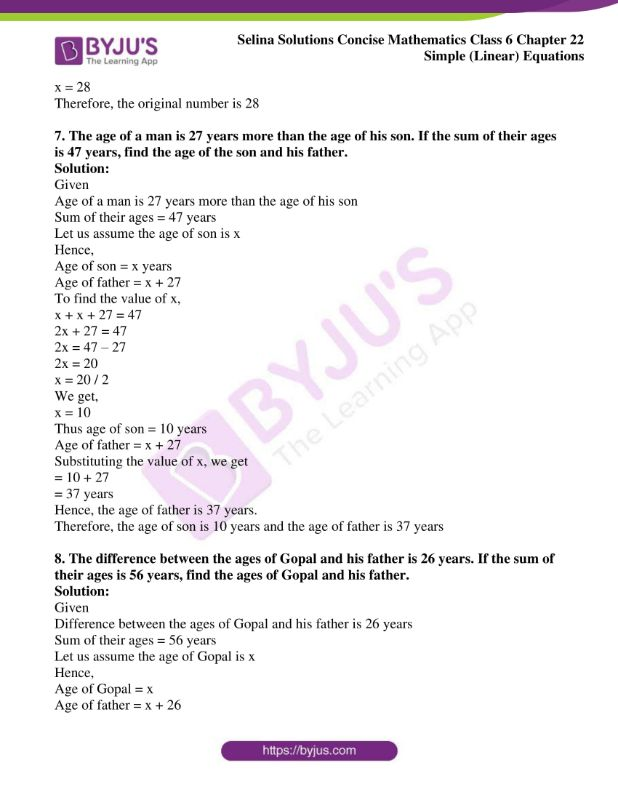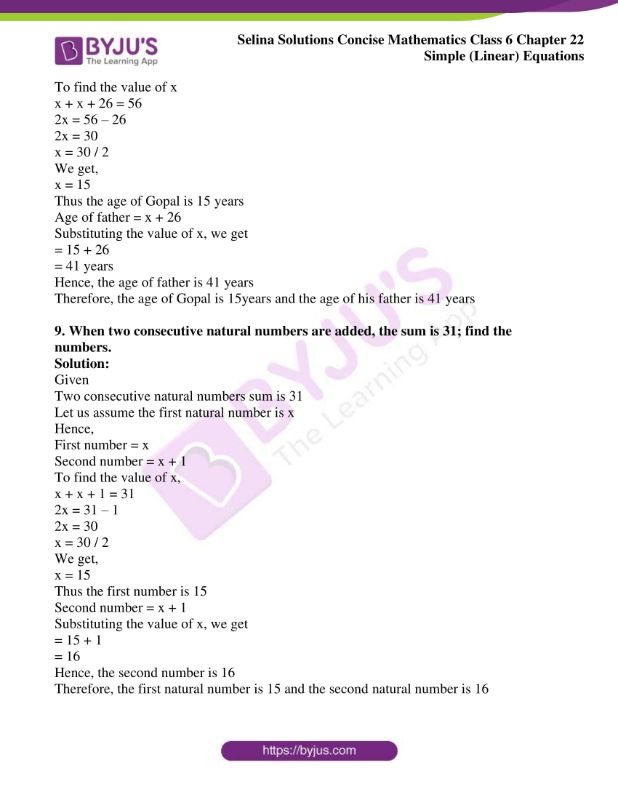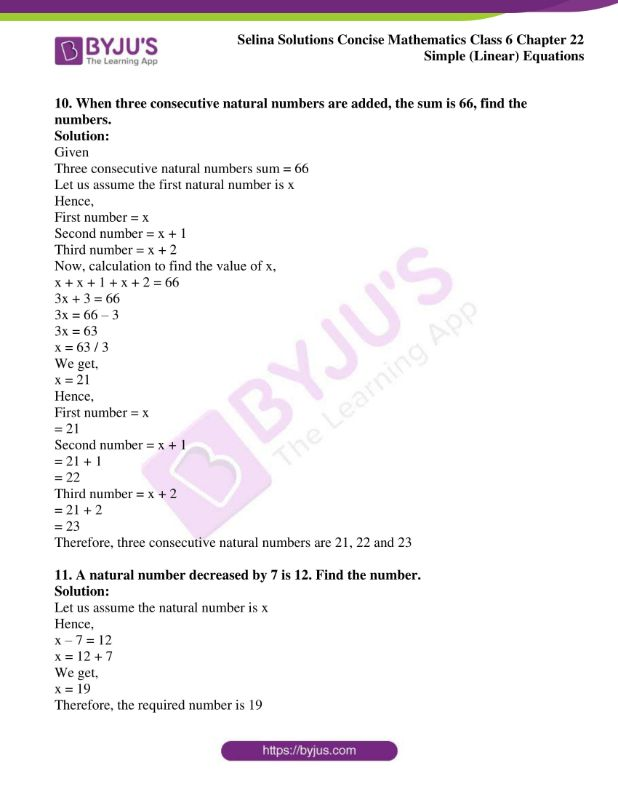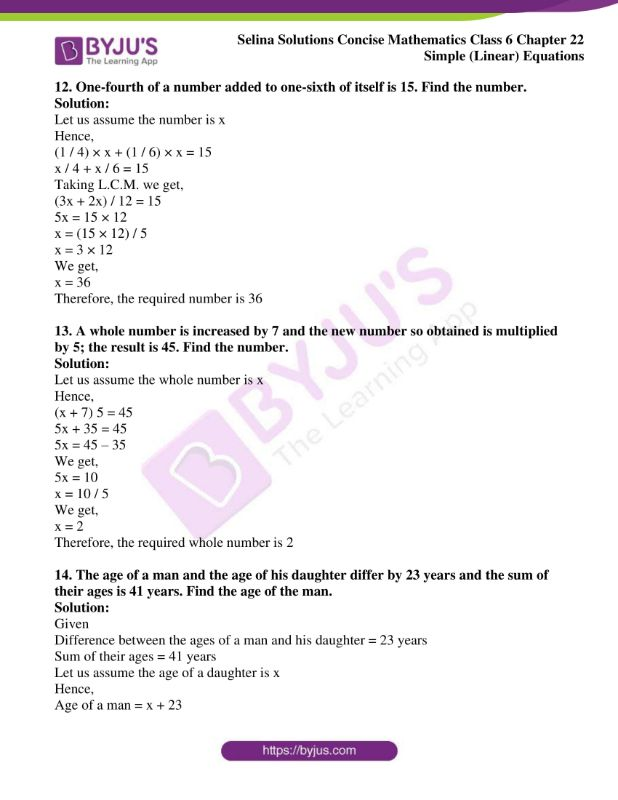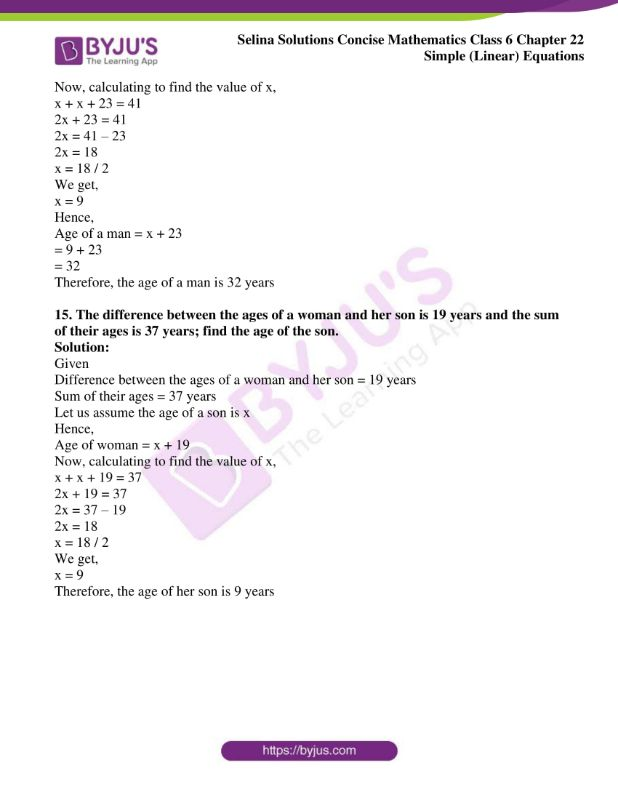### Access Selina Solutions Concise Mathematics Class 6 Chapter 22 Simple (Linear) Equations Exercise 22(D)

Exercise 22(D)

1. A number increased by 17 becomes 54. Find the number.

Solution:

Let us assume the number is x

Hence,

x + 17 = 54

x = 54 – 17

We get,

x = 37

Therefore, the required number is 37

2. A number decreased by 8 equals 26, find the number.

Solution:

Let us assume the number is x

Hence,

x – 8 = 26

x = 26 + 8

We get,

x = 34

Therefore, the required number is 34

3. One-fourth of a number add to two-seventh of it gives 135; find the number.

Solution:

Let us assume the number is x

Hence,

(1 / 4) × x + (2 / 7) × x = 135

(x / 4) + (2x / 7) = 135

Taking L.C.M. we get,

(7x + 8x) / 28 = 135

15x = 135 × 28

x = (135 × 28) / 15

We get,

x = 9 × 28

x = 252

Therefore, the required number is 252

4. Two-fifths of a number subtracted from three-fourths of it gives 56, find the number.

Solution:

Let us assume the number is x

(3 / 4) x – (2 / 5) x = 56

(3x / 4) – (2x / 5) = 56

Taking L.C.M. we get,

(15x – 8x) / 20 = 56

7x = 56 × 20

x = (56 × 20) / 7

We get,

x = 8 × 20

x = 160

Therefore, the required number is 160

5. A number is increased by 12 and the new number obtained is multiplied by 5. If the resulting number is 95, find the original number.

Solution:

Let us assume the number is x

Hence,

(x + 12) 5 = 95

5x + 60 = 95

5x = 95 – 60

5x = 35

x = 35 / 5

We get,

x = 7

Therefore, the original number is 7

6.A number is increased by 26 and the new number obtained is divided by 3. If the resulting number is 18; find the original number.

Solution:

Let us assume the number is x

Hence,

(x + 26) ÷ 3 = 18

This can be written as,

(x + 26) / 3 = 18

(x + 26) = 18 × 3

x + 26 = 54

x = 54 – 26

We get,

x = 28

Therefore, the original number is 28

7. The age of a man is 27 years more than the age of his son. If the sum of their ages is 47 years, find the age of the son and his father.

Solution:

Given

Age of a man is 27 years more than the age of his son

Sum of their ages = 47 years

Let us assume the age of son is x

Hence,

Age of son = x years

Age of father = x + 27

To find the value of x,

x + x + 27 = 47

2x + 27 = 47

2x = 47 – 27

2x = 20

x = 20 / 2

We get,

x = 10

Thus age of son = 10 years

Age of father = x + 27

Substituting the value of x, we get

= 10 + 27

= 37 years

Hence, the age of father is 37 years.

Therefore, the age of son is 10 years and the age of father is 37 years

8. The difference between the ages of Gopal and his father is 26 years. If the sum of their ages is 56 years, find the ages of Gopal and his father.

Solution:

Given

Difference between the ages of Gopal and his father is 26 years

Sum of their ages = 56 years

Let us assume the age of Gopal is x

Hence,

Age of Gopal = x

Age of father = x + 26

To find the value of x

x + x + 26 = 56

2x = 56 – 26

2x = 30

x = 30 / 2

We get,

x = 15

Thus the age of Gopal is 15 years

Age of father = x + 26

Substituting the value of x, we get

= 15 + 26

= 41 years

Hence, the age of father is 41 years

Therefore, the age of Gopal is 15years and the age of his father is 41 years

9. When two consecutive natural numbers are added, the sum is 31; find the numbers.

Solution:

Given

Two consecutive natural numbers sum is 31

Let us assume the first natural number is x

Hence,

First number = x

Second number = x + 1

To find the value of x,

x + x + 1 = 31

2x = 31 – 1

2x = 30

x = 30 / 2

We get,

x = 15

Thus the first number is 15

Second number = x + 1

Substituting the value of x, we get

= 15 + 1

= 16

Hence, the second number is 16

Therefore, the first natural number is 15 and the second natural number is 16

10. When three consecutive natural numbers are added, the sum is 66, find the numbers.

Solution:

Given

Three consecutive natural numbers sum = 66

Let us assume the first natural number is x

Hence,

First number = x

Second number = x + 1

Third number = x + 2

Now, calculation to find the value of x,

x + x + 1 + x + 2 = 66

3x + 3 = 66

3x = 66 – 3

3x = 63

x = 63 / 3

We get,

x = 21

Hence,

First number = x

= 21

Second number = x + 1

= 21 + 1

= 22

Third number = x + 2

= 21 + 2

= 23

Therefore, three consecutive natural numbers are 21, 22 and 23

11. A natural number decreased by 7 is 12. Find the number.

Solution:

Let us assume the natural number is x

Hence,

x – 7 = 12

x = 12 + 7

We get,

x = 19

Therefore, the required number is 19

12. One-fourth of a number added to one-sixth of itself is 15. Find the number.

Solution:

Let us assume the number is x

Hence,

(1 / 4) × x + (1 / 6) × x = 15

x / 4 + x / 6 = 15

Taking L.C.M. we get,

(3x + 2x) / 12 = 15

5x = 15 × 12

x = (15 × 12) / 5

x = 3 × 12

We get,

x = 36

Therefore, the required number is 36

13. A whole number is increased by 7 and the new number so obtained is multiplied by 5; the result is 45. Find the number.

Solution:

Let us assume the whole number is x

Hence,

(x + 7) 5 = 45

5x + 35 = 45

5x = 45 – 35

We get,

5x = 10

x = 10 / 5

We get,

x = 2

Therefore, the required whole number is 2

14. The age of a man and the age of his daughter differ by 23 years and the sum of their ages is 41 years. Find the age of the man.

Solution:

Given

Difference between the ages of a man and his daughter = 23 years

Sum of their ages = 41 years

Let us assume the age of a daughter is x

Hence,

Age of a man = x + 23

Now, calculating to find the value of x,

x + x + 23 = 41

2x + 23 = 41

2x = 41 – 23

2x = 18

x = 18 / 2

We get,

x = 9

Hence,

Age of a man = x + 23

= 9 + 23

= 32

Therefore, the age of a man is 32 years

15. The difference between the ages of a woman and her son is 19 years and the sum of their ages is 37 years; find the age of the son.

Solution:

Given

Difference between the ages of a woman and her son = 19 years

Sum of their ages = 37 years

Let us assume the age of a son is x

Hence,

Age of woman = x + 19

Now, calculating to find the value of x,

x + x + 19 = 37

2x + 19 = 37

2x = 37 – 19

2x = 18

x = 18 / 2

We get,

x = 9

Therefore, the age of her son is 9 years

#### 1 Comment

1. Akash deep

Hi this help me more than any apps from Google so I suggest this app isbest for students just like me